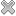# Courses / Module

Toggle Print

##DIFFERENTIAL EQUATIONS ANDĀTRANSFORMĀMETHODSĀ

Module code: EE206FZ
Credits: 5
Semester: 1
Department: INTERNATIONAL ENGINEERING COLLEGE
International:Overview

Ģ Differential equations
Ģ Review of Basic Differentiation techniques and an introduction to modelling.
Ģ Second-order differential equations
Ģ Linear constant coefficient equations. Solution using method of variation of parameters and method of undetermined coefficients.
Ģ Application to RLC series circuit and spring-mass systems.
Ģ Higher order linear differential equations.
Ģ Laplace Transform
Ģ Introduction to the Laplace transform. Idea of a linear operator. Rationale for solution method for differential equations.
Ģ Inverse Laplace Transform and its relation to the solution to a differential equation.
Ģ Laplace transforms of the elementary functions.
Ģ Shifting theorems: s-shifting and t-shifting.
Ģ Applying the Laplace transform to solving first and second-order differential equations with elementary forcing functions.
Ģ Introduction to the unit step function and its use in modelling switching functions.
Ģ Introduction to the Dirac delta function and its use in modelling impulsive functions.
Ģ Applying the Laplace transform to solving first and second-order differential equations with non-trivial forcing functions (switching, impulsive, periodic).
Ģ Laplace transform of periodic functions (square waves, triangular waves) and solving differential equations with forcing functions of this type.
Ģ Laplace transform of integrals.
Ģ Convolution theorem. Solving integral equations using the convolution theorem and their relation to time-delay systems.
Ģ Fourier Series
Ģ Introduction to Fourier series and its importance in applications.
Ģ Derivation of the Fourier coefficients.
Ģ Calculation of Fourier series for several types of periodic function.
Ģ Development and motivation of the Fourier transform.
Ģ Calculation of the Fourier Transform for various and special functions
Ģ Relation of the Fourier transform to signal analysis.
Ģ Z-Transform
Ģ Introduction to simple difference equations. Idea that solution is a sequence.
Ģ Motivational examples of how difference equations arise in practice.
Ģ Solution techniques for simple linear first and second order difference equations.
Ģ Introduction to discrete transforms and specifically the Z-transform.
Ģ Calculation of the Z-transform of certain special sequences. (Unit Step sequence, Unit Impulse sequence)
Ģ Using the Z-transform to solve difference equations and the analogy between this method and the use of the Laplace Transform.Learning OutcomesTeaching & Learning methodsAssessmentRepeat optionsTimetable